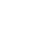# Save Python plots into Excel file

Sharing is caring!

Last Updated on July 14, 2022 by Jay

We briefly talked about how to create a graph in Python using web data, but it’s not so useful if all we can do is showing a plot in Python. What if our audience doesn’t know how to run Python and re-produce the plot? My favorite solution is to use Excel as the medium for presenting results, because nowadays, most people have Excel on their computers. So, we’ll simply save the Python generated plots into an Excel file and send the spreadsheet to the audience!

This tutorial is part of the “Integrate Python with Excel” series, you can find the table of content here for easier navigation.

Following from the previous example of plotting graphs in Python, in this post we will: 1) prettify the graph, and 2) save it into an Excel file.

## Prettify the graph

Previously we generated this graph. Although not too shabby for what a 2-line code did, the graph is far away from a professional level graph. So let’s make it prettier.

We’ll use matplotlib to modify the plot format. I won’t go through the below code since that’s not the topic of this tutorial. I will cover it in another post.

``````import pandas as pd
import matplotlib.pyplot as plt
from matplotlib.ticker import (
FormatStrFormatter,
AutoMinorLocator,
FuncFormatter,
)

import matplotlib.dates as mdates
from matplotlib.dates import DateFormatter

df = df.iloc[:,4:]
global_num = df.sum()

fig, ax = plt.subplots()
ax.set(title = 'Global Covid Confirmed Cases',
xlabel = 'Time',
ylabel = 'Number of cases')

ax.yaxis.set_major_formatter(FuncFormatter(lambda x, p: format(x/1000000) +'M'))

date_form = DateFormatter("%m-%d")
ax.xaxis.set_major_formatter(date_form)
locator = mdates.DayLocator()
ax.xaxis.set_major_locator(locator)

ax.xaxis.set_major_locator(mdates.WeekdayLocator(interval=3))
ax.yaxis.grid()
dates = pd.to_datetime(global_num.index)
plt.plot(dates, global_num)
plt.show()``````

## Save a Python generated plot into Excel file

We’ll need to save the plot to our computer first.

``plt.savefig('python_pretty_plot.png')``

Then we can use xlsxwriter library to create an Excel file! To save the confirmed cases data into Excel:

``````writer = pd.ExcelWriter('python_plot.xlsx', engine = 'xlsxwriter')
global_num.to_excel(writer, sheet_name='Sheet1')
``````

Then we can add the image to the same sheet:

``````worksheet = writer.sheets['Sheet1']
worksheet.insert_image('C2','python_pretty_plot.png')
writer.save()``````

1.Terence Cosgrove says:

Can you do this without first writing file eg using IoBytes- my attempts so far have failed

1.Jay says:

Since we are using the xlsxwriter module, apparently in the worksheet.insert_image() method there’s another argument “options”, and we can pass a bytesio object (which contains a plt plot) there… Also make sure you save the workbook before closing the bytesio.

Try this:

import matplotlib.pyplot as plt
import pandas as pd
import io

x = [1,2,3,4,5]
y = [2,4,6,8,10]
fig = plt.figure()
plt.plot(x,y)
plt.title(‘in memory’)
writer = pd.ExcelWriter(‘python_plot.xlsx’, engine = ‘xlsxwriter’)
df=pd.DataFrame({‘a’:[1,2,3,4]}).to_excel(writer, sheet_name=”Sheet1″) #write some random data

buf = io.BytesIO()
plt.savefig(buf, format=’png’)
buf.seek(0)
worksheet = writer.sheets[‘Sheet1’]
worksheet.insert_image(‘C2’, ‘a’, options={‘image_data’:buf}) #’a’ is the name of the image which doesn’t matter
writer.save()
buf.close()